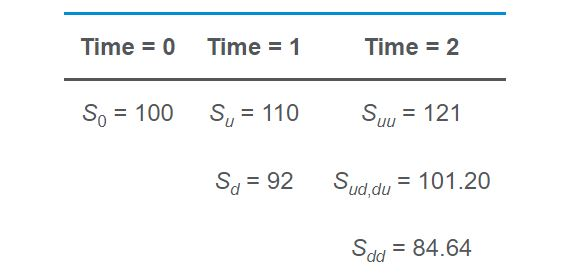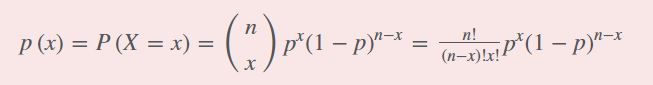# Question on Binomial Tree

Assume that a stock’s price over the next two periods is as shown below.The initial value of the stock is \$100. The probability of an up move in any given period is 40%, and the probability of a down move in any given period is 60%. Using the binomial model, the probability that the stock’s price will be \$101.20 at the end of two periods is closest to:

1. 48%.
2. 24%.
3. 16%.where

n = 2 (number of periods)

x = 1 (number of up moves: ud and du)

p = 0.40 (probability of an up move)

p(1)=(21)0.401(1−0.40)2−1=2!(2−1)!1!×0.401×0.61=2×0.40×0.60=0.48

Can somebody help me understand why ‘x (number of up moves)’ is 1?

Their notation leaves a bit to be desired. The middle entry in the last column should read,

S_{ud} = S_{du} = 101.20

and their explanation should read, in part,

x = 1 (number of up moves: ud or du)

1 Like

It’s virtually the same as flipping a coin twice and asking for the probability of getting 1 tail. For the stock question, all you care about is you had 1 u and 1 d: you don’t care in which order it happened.

1 Like

Ah, I was getting confused since it said ud and du.
Thank you!Thank You that helped!1 Like